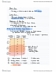# ECO101H1 Lecture Notes - Lecture 4: Shortage, Potential Output, Autonomous Consumption

25 views6 pages
School
Department
Course
Professorthaany10 and 37865 others unlocked98
Verified Note
98 documents

## Document Summary

Equilibrium income : y = ae at yeq: if ae > y: desired > actual (excess demand, if ae < y: desired < actual (excess supply) The simple model: no government (g=0), no trade (x=m=0) : > c + i = y at yeq: consumption function (c): total desired personal consumption, investment function (i): total desired investment. Spend all disposable income (yd) on either consumption ( c ) or saving ( s ) Marginal propensity to consume (mpc) [slope of cs] Yd = c + s and c = c( + mpcyd. Therefore, s = - c( + (1-mpc) yd. Equilibrium consumption : c = yd (see lec 4 p23 ex. ) Consider investment as inventories: if actual sales < planned sales: actual inventories > planned. Inventories -> firms respond by reducing production: if actual sales > planned sales: actual inventories < planned. In equilibrium: ae = y at yeq (i. e. desired expenditure equals actual output)